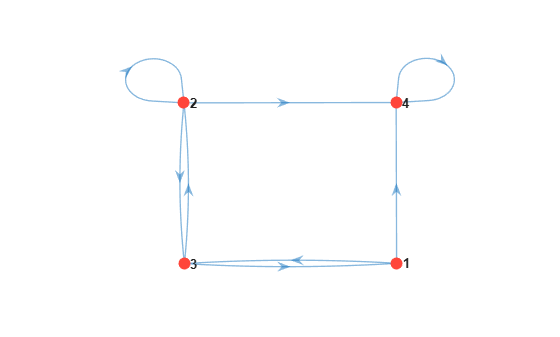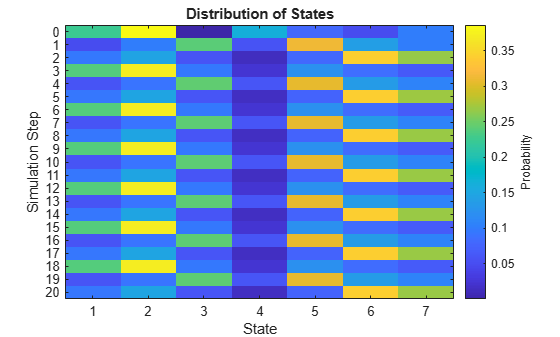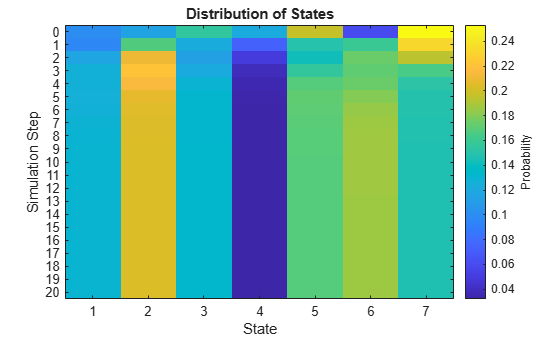# redistribute

Compute Markov chain redistributions

## Syntax

``X = redistribute(mc,numSteps)``
``X = redistribute(mc,numSteps,'X0',x0)``

## Description

example

``X = redistribute(mc,numSteps)` returns data `X` on the evolution of a uniform distribution of states in the discrete-time Markov chain `mc` after it advances `numSteps` time steps.`

example

``X = redistribute(mc,numSteps,'X0',x0)` optionally specifies the initial state distribution `x0`.`

## Examples

collapse all

Create a four-state Markov chain from a randomly generated transition matrix containing eight infeasible transitions.

```rng('default'); % For reproducibility mc = mcmix(4,'Zeros',8);```

`mc` is a `dtmc` object.

Plot a digraph of the Markov chain.

```figure; graphplot(mc);```State `4` is an absorbing state.

Compute the state redistributions at each step for 10 discrete time steps. Assume an initial uniform distribution over the states.

`X = redistribute(mc,10)`
```X = 11×4 0.2500 0.2500 0.2500 0.2500 0.0869 0.2577 0.3088 0.3467 0.1073 0.2990 0.1536 0.4402 0.0533 0.2133 0.1844 0.5489 0.0641 0.2010 0.1092 0.6257 0.0379 0.1473 0.1162 0.6985 0.0404 0.1316 0.0765 0.7515 0.0266 0.0997 0.0746 0.7991 0.0259 0.0864 0.0526 0.8351 0.0183 0.0670 0.0484 0.8663 ⋮ ```

`X` is an 11-by-4 matrix. Rows correspond to time steps, and columns correspond to states.

Visualize the state redistribution.

```figure; distplot(mc,X)```After 10 transitions, the distribution appears to settle with a majority of the probability mass in state `4`.

Consider this theoretical, right-stochastic transition matrix of a stochastic process.

`$P=\left[\begin{array}{ccccccc}0& 0& 1/2& 1/4& 1/4& 0& 0\\ 0& 0& 1/3& 0& 2/3& 0& 0\\ 0& 0& 0& 0& 0& 1/3& 2/3\\ 0& 0& 0& 0& 0& 1/2& 1/2\\ 0& 0& 0& 0& 0& 3/4& 1/4\\ 1/2& 1/2& 0& 0& 0& 0& 0\\ 1/4& 3/4& 0& 0& 0& 0& 0\end{array}\right].$`

Create the Markov chain that is characterized by the transition matrix P.

```P = [ 0 0 1/2 1/4 1/4 0 0 ; 0 0 1/3 0 2/3 0 0 ; 0 0 0 0 0 1/3 2/3; 0 0 0 0 0 1/2 1/2; 0 0 0 0 0 3/4 1/4; 1/2 1/2 0 0 0 0 0 ; 1/4 3/4 0 0 0 0 0 ]; mc = dtmc(P);```

Plot a directed graph of the Markov chain. Indicate the probability of transition by using edge colors.

```figure; graphplot(mc,'ColorEdges',true);```Compute a 20-step redistribution of the Markov chain using random initial values.

```rng(1); % For reproducibility x0 = rand(mc.NumStates,1); rd = redistribute(mc,20,'X0',x0);```

Plot the redistribution.

```figure; distplot(mc,rd);```The redistribution suggests that the chain is periodic with a period of three.

Remove periodicity by creating a lazy version of the Markov chain.

`lc = lazy(mc);`

Compute a 20-step redistribution of the lazy chain using random initial values. Plot the redistribution.

```x0 = rand(mc.NumStates,1); lrd1 = redistribute(lc,20,'X0',x0); figure; distplot(lc,lrd1);```The redistribution appears to settle after several steps.

## Input Arguments

collapse all

Discrete-time Markov chain with `NumStates` states and transition matrix `P`, specified as a `dtmc` object. `P` must be fully specified (no `NaN` entries).

Number of discrete time steps to compute, specified as a positive integer.

Data Types: `double`

### Name-Value Pair Arguments

Specify optional comma-separated pairs of `Name,Value` arguments. `Name` is the argument name and `Value` is the corresponding value. `Name` must appear inside quotes. You can specify several name and value pair arguments in any order as `Name1,Value1,...,NameN,ValueN`.

Example: `'X0',[0.5 0.25 0.25]` specifies an initial state distribution of `[0.5 0.25 0.25]`.

Initial distribution, specified as the comma-separated pair consisting of `'X0'` and a nonnegative numeric vector of `NumStates` length. `redistribute` normalizes `X0` so that it sums to `1`.

The default is a uniform distribution of states.

Example: `'X0',[0.5 0.25 0.25]`

Data Types: `double`

## Output Arguments

collapse all

Evolution of state probabilities, returned as a `(1 + numSteps)`-by-`NumStates` nonnegative numeric matrix. The first row is `X0`. Subsequent rows are the redistributions at each step, which `redistribute` determines by the transition matrix `P`.

Note

If `mc` is ergodic, and `numSteps` is sufficiently large, `X(end,:)` approximates `x = asymptotics(mc)`. See `asymptotics`.

## Tips

To visualize the data created by `redistribute`, use `distplot`.

### Functions

Introduced in R2017b